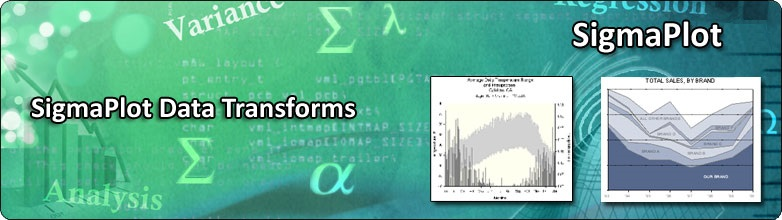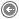DATA TRANSFORMATION
 SigmaPlot comes with a simple, easy-to-use scripting language for manipulating data in an active worksheet. The scripting language can allocate memory to variables; perform looping, Boolean logic, and evaluate functions to transform a worksheet. Worksheet transforms can be entered into either the Quick Transform dialog, or the User-Defined Transform dialog. New users will find the Quick Transform dialog to be a gradual introduction to single line transforms.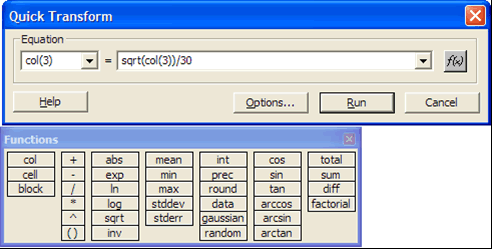Experienced and more demanding users will find the User-Defined Transform dialog to be a powerful way to transform worksheet data. As of SigmaPlot 14, user-defined transforms can be stored as an item in a notebook along with the data.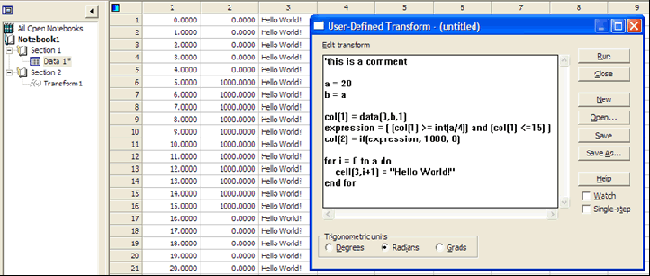The following list groups transforms by function type. It is followed by an alphabetical reference containing complete descriptions of all transform functions and their syntax, with examples. Worksheet Functions These worksheet functions are used to specify cells and columns from the worksheet, either to read data from the worksheet for transformation, or to specify a destination for transform results. Function Description block The block function returns a specified block of cells from the worksheet. block height & width The blockheight and blockwidth functions return a specified block of cells or block dimension from the worksheet. cell The cell function returns a specific cell from the worksheet. col The col function returns a worksheet column or portion of a column. put into The put into function places variable or equation results in a worksheet column. subblock The subblock function returns a specified block of cells from within another block. Data Manipulation Function The data manipulation functions are used to generate non-random data, and to sample, select, and sort data. Function Description data The data function generates serial data. if The if function conditionally selects between two data sets. nth The nth function returns an incremental sampling of data. sort The sort function rearranges data in ascending order. Trigonometrics Function SigmaPlot and SigmaStat provide a complete set of trigonometric functions. Function Description arccos This function returns the arccosine of the specified argument. arcsin This function returns the arcsine of the specified argument. arctan This function returns the arctangent of the specified argument. cos This function returns the cosine of the specified argument. sin This function returns the sine of the specified argument. tan This function returns the tangent of the specified argument. cosh This function returns the hyperbolic cosine of the specified argument. sinh This function returns the hyperbolic sine of the specified argument. tanh This function returns the hyperbolic tangent of the specified argument. Numeric Functions The numeric functions perform a specific type of calculation on a number or range of numbers and returns the appropriate results. Function Description abs The abs function returns the absolute value. exp The exp function returns the values for e raised to the specified numbers. factorial The factorial function returns the factorial for each specified number. mod The mod function returns the modulus, or remainder of division, for specified numerators and divisors. In The ln function returns the natural logarithm for the specified numbers. log The log function returns the base 10 logarithm for the specified numbers. sqrt The sqrt function returns the square root for the specified numbers. Range Functions The following functions give information on ranges. Function Description count The count function returns the number of numeric values in a range. missing The missing function returns the number of missing values and text strings in a range. size The size function returns the number of data points in a range, including all numbers, missing values, and text strings. Accumulation Function The accumulation functions return values equal to the accumulated operation of the function. Function Description diff The diff function returns the differences of the numbers in a range. sum The sum function returns the cumulative sum of a range of numbers. total The total function returns the value of the total sum of a range. Random Generation Function The two “random” number generating functions can be used to create a series of normally or uniformly distributed numbers. Function Description gaussian The Gaussian function is used to generate a series of normally (Gaussian or “bell” shaped) distributed numbers with a specified mean and standard deviation. random The random function is used to generate a series of uniformly distributed numbers within a specified range. Precision Functions The precision functions are used to convert numbers to whole numbers or to round off numbers. Function Description int The int function converts numbers to integers. prec The prec function rounds numbers off to a specified number of significant digits. round The round function rounds numbers off to a specified number of decimal places. Statistical Functions The statistical functions perform statistical calculations on a range or ranges of numbers. Function Description avg The avg function calculates the averages of corresponding numbers across ranges. It can be used to calculate the average across rows for worksheet columns. max The max function returns the largest value in a range. min The min function returns the smallest value in a range. mean The mean function calculates the mean of a range. runavg The runavg function produces a range of running averages. stddev The stddev function returns the standard deviation of a range. stderr The stderr function calculates the standard error of a range. avg The avg function calculates the averages of corresponding numbers across ranges. It can be used to calculate the average across rows for worksheet columns. max The max function returns the largest value in a range. min The min function returns the smallest value in a range. Area and Distance Function These functions can be used to calculate the areas and distances specified by X,Y coordinates. Units are based on the units used for X and Y. Function Description area The area function finds the area of a polygon described in X,Y coordinates. distance The distance function calculates the distance of a line whose segments are described in X,Y coordinates. partdist The partdist function calculates the distances from an initial X,Y coordinate to successive X,Y coordinates in a cumulative fashion. Curve Fitting Function These functions are designed to be used in conjunction with SigmaPlot’s nonlinear curve fitter, to allow automatic determination of initial equation parameter estimates from the source data. You can use these functions to develop your own parameter determination function by using the functions provided with the Standard Regression Equations library provided with SigmaPlot. Function Description ape This function is used for the polynomials, rational polynomials and other functions which can be expressed as linear functions of the parameters. A linear least squares estimation procedure is used to obtain the parameter estimates. dsinp This function returns an estimate of the phase in radians of damped sine functions. fwhm This function returns the x width of a peak at half the peak’s maximum value for peak shaped functions. inv The inv function generates the inverse matrix of an invertible square matrix provided as a block. lowess The lowess algorithm is used to smooth noisy data. “Lowess” means locally weighted regression. Each point along the smooth curve is obtained from a regression of data points close to the curve point with the closest points more heavily weighted. lowpass The lowpass function returns smoothed y values from ranges of x and y variables, using an optional user-defined smoothing factor that uses FFT and IFFT. sinp This function returns an estimate of the phase in radians of sinusoidal functions. x25 This function returns the x value for the y value 25% of the distance from the minimum to the maximum of smoothed data for sigmoidal shaped functions. x50 This function returns the x value for the y value 50% of the distance from the minimum to the maximum of smoothed data for sigmoidal shaped functions. x75 This function returns the x value for the y value 75% of the distance from the minimum to the maximum of smoothed data for sigmoidal shaped functions. xatxmax This function returns the x value for the maximum y in the range of y coordinates for peak shaped functions. xwtr This function returns x75-x25 for sigmoidal shaped functions. Miscellaneous Functions These functions are specialized functions which perform a variety of operations. Function Description choose The choose function is the mathematical “n choose r” function. histogram The histogram function generates a histogram from a range or column of data. interpolate The interpolate function performs linear interpolation between X,Y coordinates. polynomial The polynomial function returns results for specified independent variables for a specified polynomial equation. rgbcolor The rgbcolor(r,g,b) color function takes arguments r,g, and b between 0 and 255 and returns color to cells in the worksheet. Special Constructs Transform constructs are special structures that allow more complex procedures than functions. Function Description for The for statement is a looping construct used for iterative processing. if... then... else The if...then...else construct proceeds along one of two possible series of procedures based on the results of a specified condition. Fast Fourier Transform Functions These functions are used to remove noise from and smooth data using frequency-based filtering. Function Description fft The fft function finds the frequency domain representation of your data. invfft The invfft function takes the inverse fft of the data produced by the fft to restore the data to its new filtered form. real The real function strips the real numbers out of a range of complex numbers. img The img function strips the imaginary numbers out of a range of complex numbers. complex The complex function converts a block of real and/or imaginary numbers into a range of complex numbers. mulcpx The mulcpx function multiplies two ranges of complex numbers together. invcpx The invcpx takes the reciprocal of a range of complex numbers.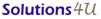MY Office : 72-3C, JALAN PUTERI 2/4, BANDAR PUTERI, 47100 PUCHONG, SELANGOR, Malaysia. Tel:+603-8063 9300 fax:+603-8063 9400 SG Office : 259, Onan Road, Singapore 424651. Tel: +65-6468 3325 Fax: +65-6764 5646